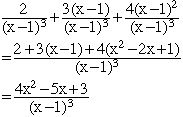Hello, I've come up with an answer for the following problem but it's not the same as the book's answer. However, the book has a lot of mistakes in it and I don't know if I'm doing it properly or not. Your help would be soooo appreciated. It's driving me crazy.The problem is: ``` 2 + 3 + 4 --------- + --------- + ---------- (x-1)^3 (x-1)^2 (x-1) ``` [(x-1)^3 is (x-1) to power of 3 etc.] Thank you Francine Hi Francine, The first chore is to get all three fractions to have a common denominator. The best choice here for that denominator is (x-1)3. To accomplish this multiply the numerator and denominator of the second fraction by (x-1) and multiply the numerator and denominator of the third fraction by (x-1)2. The result isThe expression in the numerator doesn't factor so there is no further simplification. Cheers, Penny Go to Math Central